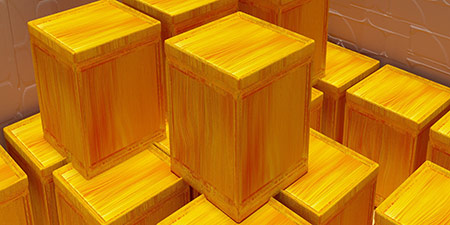# Cubic Meter (m³) to Liter (l)

Volume ﹣ Converter Cubic Meter to Liter

Here you can convert the Volume unit Cubic Meter into the unit Liter and vice versa you can convert Liter into Cubic Meter. By clicking the "Swap units" icon, you will always obtain the desired conversion in the calculation result, i.e., m³ to l or l to m³. With the following calculator you can also calculate any other Volume unit.

One cubic meter (m³; spelled metre in Commonwealth English and meter in American English) corresponds to the volume of a cube with an edge length of one meter.

1 m³ = 1 m × 1 m × 1 m

The cubic meter is the base unit for volume in the International System of Units (SI) and in other metric systems. All SI-compliant units of volume are derived from the metre and its parts and multiples.

One liter (l; spelled litre in Commonwealth English and liter in American English) corresponds to one cubic decimeter (dm³) and thus to the volume of a cube with an edge length of 10 cm.

The liter is not part of the International System of Units (SI), but is approved for use with the SI. In addition, the liter is a legal unit of measurement due to national laws.

## Basis for conversion Cubic Meter (m³) to Liter (l) and vice versa

The abbreviation for the "Volume unit Cubic Meter" is m³. The abbreviation for the "Volume unit Liter" is l.

## Formula for the conversion of Cubic Meter (m³) to Liter (l) and vice versa

The calculation from Cubic Meter to Liter shall be made using the following conversion formula:

Conversion formula Cubic Meter to Liter

Determine the number of Liter from Cubic Meter

Cubic Meter × 1000

## Formula for the conversion of Liter (l) to Cubic Meter (m³)

The calculation from Liter to Cubic Meter shall be made using the following conversion formula:

Conversion formula Liter to Cubic Meter

Determine the number of Cubic Meter from Liter

Liter × 0.001

## Overview table : How many Cubic Meter are in a Liter ?

Cubic Meter m³ ⇒ Liter l
0.01 m³  are  10 l
0.02 m³  are  20 l
0.03 m³  are  30 l
0.04 m³  are  40 l
0.05 m³  are  50 l
0.06 m³  are  60 l
0.07 m³  are  70 l
0.08 m³  are  80 l
0.09 m³  are  90 l
0.10 m³  are  100 l
0.20 m³  are  200 l
0.30 m³  are  300 l
0.40 m³  are  400 l
0.50 m³  are  500 l
0.60 m³  are  600 l
0.70 m³  are  700 l
0.80 m³  are  800 l
0.90 m³  are  900 l
1 m³  corresponds to  1 000 l
2 m³  are  2 000 l
3 m³  are  3 000 l
4 m³  are  4 000 l
5 m³  are  5 000 l
6 m³  are  6 000 l
7 m³  are  7 000 l
8 m³  are  8 000 l
9 m³  are  9 000 l
10 m³  are  10 000 l
20 m³  are  20 000 l
30 m³  are  30 000 l
40 m³  are  40 000 l
50 m³  are  50 000 l
60 m³  are  60 000 l
70 m³  are  70 000 l
80 m³  are  80 000 l
90 m³  are  90 000 l
100 m³  are  100 000 l
200 m³  are  200 000 l
300 m³  are  300 000 l
400 m³  are  400 000 l
500 m³  are  500 000 l
600 m³  are  600 000 l
700 m³  are  700 000 l
800 m³  are  800 000 l
900 m³  are  900 000 l
1 000 m³  are  1 000 000 l

## Overview table : How many Liter are in a Cubic Meter ?

Liter l ⇒ Cubic Meter m³
0.01 l  are  0.00001 m³
0.02 l  are  0.00002 m³
0.03 l  are  0.00003 m³
0.04 l  are  0.00004 m³
0.05 l  are  0.00005 m³
0.06 l  are  0.00006 m³
0.07 l  are  0.00007 m³
0.08 l  are  0.00008 m³
0.09 l  are  0.00009 m³
0.10 l  are  0.00010 m³
0.20 l  are  0.00020 m³
0.30 l  are  0.00030 m³
0.40 l  are  0.00040 m³
0.50 l  are  0.00050 m³
0.60 l  are  0.00060 m³
0.70 l  are  0.00070 m³
0.80 l  are  0.00080 m³
0.90 l  are  0.00090 m³
1 l  corresponds to  0.00100 m³
2 l  are  0.00200 m³
3 l  are  0.00300 m³
4 l  are  0.00400 m³
5 l  are  0.00500 m³
6 l  are  0.00600 m³
7 l  are  0.00700 m³
8 l  are  0.00800 m³
9 l  are  0.00900 m³
10 l  are  0.01000 m³
20 l  are  0.02000 m³
30 l  are  0.03000 m³
40 l  are  0.04000 m³
50 l  are  0.05000 m³
60 l  are  0.06000 m³
70 l  are  0.07000 m³
80 l  are  0.08000 m³
90 l  are  0.09000 m³
100 l  are  0.10000 m³
200 l  are  0.20000 m³
300 l  are  0.30000 m³
400 l  are  0.40000 m³
500 l  are  0.50000 m³
600 l  are  0.60000 m³
700 l  are  0.70000 m³
800 l  are  0.80000 m³
900 l  are  0.90000 m³
1 000 l  are  1 m³

## Source information

As source for the information in the 'Volume' category, we have used in particular:

## Last update

This page of the 'Volume' category was last edited or reviewed by Stefan Banse on February 20, 2023. It corresponds to the current status.

### Changes in this category "Volume"

• 28.11.2022: Publication of the topic Convert Volumes together with the corresponding texts.
• 28.11.2022: Extension of the Volume Converter by further units: Liquid ounce, cup, tablespoon and teaspoon